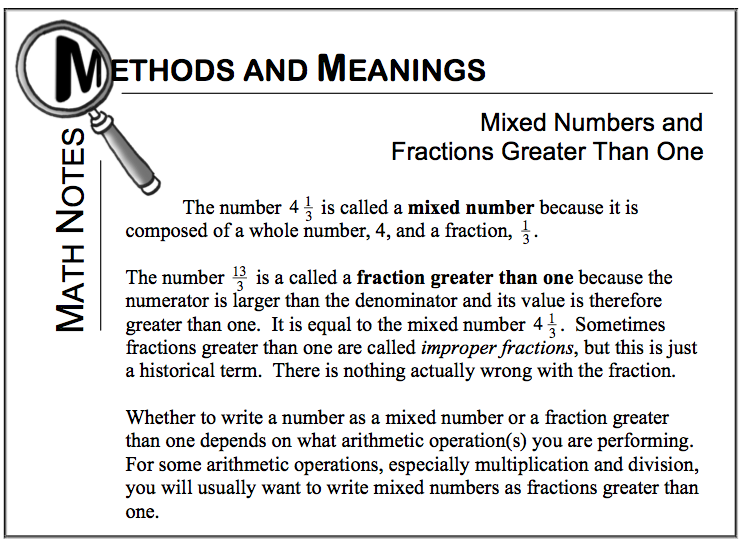Home > CC2MN > Chapter cc22 > Lesson cc22.1.1 > Problem2-17

2-17.

Read the Math Notes box for this lesson. Then rewrite each number below as a single fraction greater than one. Homework Help ✎

1. $1\frac{1}{11}$

If $1=\frac{11}{11}$$1+\frac{1}{11}=\frac{?}{11}$

$\frac{12}{11}$

1. $3\frac{2}{5}$

Use a similar pattern to find part (b).

1. $2\frac{4}{15}$

Use a similar pattern to find part (c).